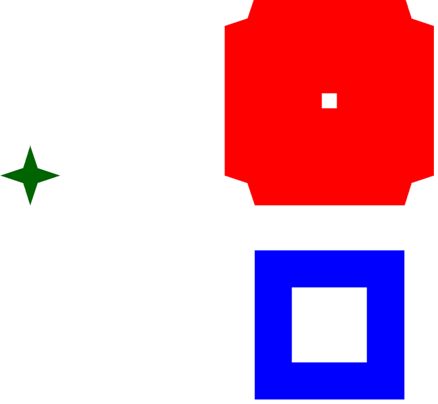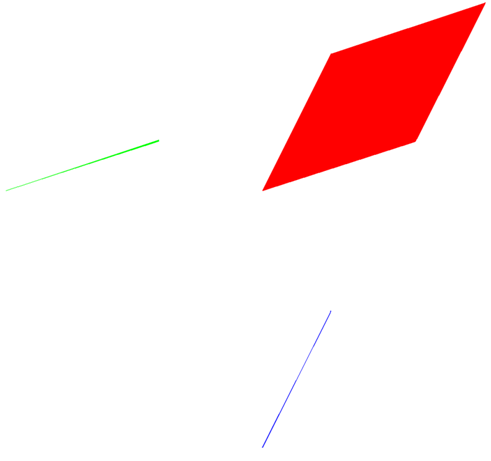# Minkowski Sum Tutorial

In this tutorial we will implement an algorithm to compute the Minkowski sum of two polygon sets.  The Minkowski sum of two polygon sets is the convolution of the two polygon sets and is defined as the set of points which is produced if you sum all pairs of points in the two polygon sets.  The sum of two points is implemented in Boost.Polygon by the convolve function for point_concept.  Similarly there is a convolve function for rectangle_concept.  The convolution of two polygon sets produces a polygon set as its output.  An example of the convolution of two polygons is shown below.  The center point of the green star can be imagined to slide around freely inside the blue picture frame shape painting the area the star touches to produce the red bloated picture frame.The above image was produced using the code presented in this tutorial.  We can see that the algorithm for Minkowski sum should support non-convex polygons that may potentially have holes.  It should also support disjoint polygons in both input polygon sets.  Shown below is what happens when multiple polygons are present in each input polygon set.In each of these examples the origin of the coordinate system is in the lower left corner of the image and the sum of the x and y locations of the input polygons places the result in the upper right hand corner of the images.  In the example above the lower left red triangle is the convolution of the small blue triangle with the small green triangle.  The upper right red triangle is the convolution of the large blue and large green triangle.  The two medium sized red polygons are the result of the convolution of the small with large and large with small blue and green triangles.  The test case was carefully contrived to prevent the result from merging together, though that could certainly happen.

The algorithm implemented for Minkowski sum in this tutorial is very simple.  It is based on the convolution of polygon edges.  The convolution of two edges is very easy to compute and is in general a parallelogram.  An example of two edges being convolved to produce a parallelogram is shown below.Now that we know what we need from a function to convolve two edges, let's implement one now.  Below we show the code for convolving two edges along with some type definitions we will be using throughout the tutorial.  The code for this tutorial is available in minkowski.cpp.

typedef boost::polygon::point_data<int> point;
typedef boost::polygon::polygon_set_data<int> polygon_set;
typedef boost::polygon::polygon_with_holes_data<int> polygon;
typedef std::pair<point, point> edge;
using namespace boost::polygon::operators;

void convolve_two_segments(std::vector<point>& figure, const edge& a, const edge& b) {
using namespace boost::polygon;
figure.clear();
figure.push_back(point(a.first));
figure.push_back(point(a.first));
figure.push_back(point(a.second));
figure.push_back(point(a.second));
convolve(figure, b.second);
convolve(figure, b.first);
convolve(figure, b.first);
convolve(figure, b.second);
}

This function for convolving two line segments just convolves the end points of the two line segments in all combinations to produce the four points of a parallelogram and populates a vector of points with them in the correct order.  We are using the Boost.Polygon library function for convolving two points and the library point data structure.

To compute the convolution of two polygon sets we start by taking the union of the convolution of all pairs of edges between the two polygon sets.  Given an operation for convolving two edges it is pretty easy to convolve all pairs of edges of two polygon sets.  The result is the convolution the perimeters of the polygon sets, but doesn't handle the convolution of their interiors.  To illustrate this we show what happens when one of the above examples is computed using just the all pairs of edges convolution.As we can see, the result is as if the small triangles were slid around the perimeter of the large triangles leaving a hole in the center that should be filled in if the small triangle were allowed to slide freely within the large triangle.  Also available in Boost.Polygon is the convolve function for polygon_concept that convolves a polygon over a point.  All this does is translate the polygon by the x and y value of the point.  To fill in the interior regions of the result of the convolution of two polygon sets we perform an all pairs of polygon convolution to the first vertex of the other polygon and merge that into the union of edge convolutions.

Let's implement the rest of Minkowski sum of two polygon sets as the union of all pairs edges convolutions and the all pairs of polygons to point convolutions.  First we implement a function that convolves all pairs of edges represented by iterator pairs over points.  We assume that the first point is equal to the last point in each sequence because we know the polygons that gave rise to the iterators were produce by the Boost.Polygon algorithm for general polygon formation.

template <typename itrT1, typename itrT2>
void convolve_two_point_sequences(polygon_set& result, itrT1 ab, itrT1 ae, itrT2 bb, itrT2 be) {
using namespace boost::polygon;
if(ab == ae || bb == be)
return;
point prev_a = *ab;
std::vector<point> vec;
polygon poly;
++ab;
for( ; ab != ae; ++ab) {
point prev_b = *bb;
itrT2 tmpb = bb;
++tmpb;
for( ; tmpb != be; ++tmpb) {
convolve_two_segments(vec, std::make_pair(prev_b, *tmpb), std::make_pair(prev_a, *ab));
set_points(poly, vec.begin(), vec.end());
result.insert(poly);
prev_b = *tmpb;
}
prev_a = *ab;
}
}

Using the function defined above we can implement a function for computing the convolution of all pairs of edges represented by an iterator pair over points and edges in a collection of polygons.  We just call the convolve_two_point_sequences for the input point sequence and all outer shell and hole point sequences from the container of polygons.

template <typename itrT>
void convolve_point_sequence_with_polygons(polygon_set& result, itrT b, itrT e, const std::vector<polygon>& polygons) {
using namespace boost::polygon;
for(std::size_t i = 0; i < polygons.size(); ++i) {
convolve_two_point_sequences(result, b, e, begin_points(polygons[i]), end_points(polygons[i]));
for(polygon_with_holes_traits<polygon>::iterator_holes_type itrh = begin_holes(polygons[i]);
itrh != end_holes(polygons[i]); ++itrh) {
convolve_two_point_sequences(result, b, e, begin_points(*itrh), end_points(*itrh));
}
}
}

Using the function defined above we can implement a function for computing the convolution of all pairs of edges from polygons contained within two polygon sets.  We also convolve each polygon with the first vertex of every polygon from the other set to fill in the interiors of the result.

void convolve_two_polygon_sets(polygon_set& result, const polygon_set& a, const polygon_set& b) {
using namespace boost::polygon;
result.clear();
std::vector<polygon> a_polygons;
std::vector<polygon> b_polygons;
a.get(a_polygons);
b.get(b_polygons);
for(std::size_t ai = 0; ai < a_polygons.size(); ++ai) {
convolve_point_sequence_with_polygons(result, begin_points(a_polygons[ai]),
end_points(a_polygons[ai]), b_polygons);
for(polygon_with_holes_traits<polygon>::iterator_holes_type itrh = begin_holes(a_polygons[ai]);
itrh != end_holes(a_polygons[ai]); ++itrh) {
convolve_point_sequence_with_polygons(result, begin_points(*itrh),
end_points(*itrh), b_polygons);
}
for(std::size_t bi = 0; bi < b_polygons.size(); ++bi) {
polygon tmp_poly = a_polygons[ai];
result.insert(convolve(tmp_poly, *(begin_points(b_polygons[bi]))));
tmp_poly = b_polygons[bi];
result.insert(convolve(tmp_poly, *(begin_points(a_polygons[ai]))));
}
}
}

We test the convolution of two polygon set with the code shown below that produces the first example shown in this tutorial.

polygon_set a, b, c;
a += boost::polygon::rectangle_data<int>(0+300, 0, 200+300, 200);
a -= boost::polygon::rectangle_data<int>(50+300, 50, 150+300, 150);
std::vector<polygon> polys;
std::vector<point> pts;
pts.push_back(point(-40, 0+300));
pts.push_back(point(-10, 10+300));
pts.push_back(point(0, 40+300));
pts.push_back(point(10, 10+300));
pts.push_back(point(40, 0+300));
pts.push_back(point(10, -10+300));
pts.push_back(point(0, -40+300));
pts.push_back(point(-10, -10+300));
pts.push_back(point(-40, 0+300));
polygon poly;
boost::polygon::set_points(poly, pts.begin(), pts.end());
b+=poly;
polys.clear();
convolve_two_polygon_sets(c, a, b);

Output (a is blue, b is green and c is red):This concludes our tutorial on how to implement Minkowski sum of polygons as the convolution of polygon sets based on the Boolean OR operation of geometry produced by simpler convolution features provided by the Boost.Polygon library.